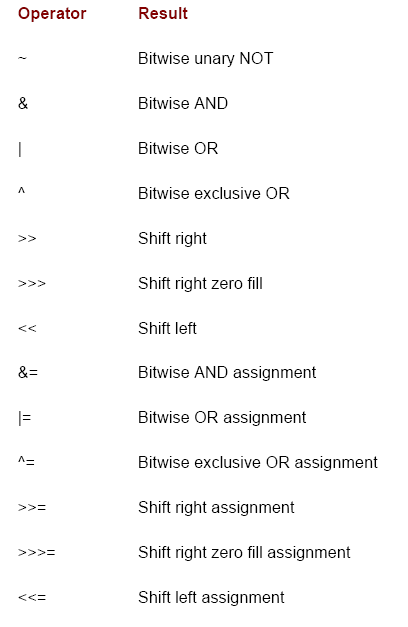# Programming Tutorials

## Bitwise operators in Java

By: Baski in Java Tutorials on 2007-09-07

Java defines several bitwise operators which can be applied to the integer types, long, int, short, char, and byte. These operators act upon the individual bits of their operands. They are summarized in the table below:

Since the bitwise operators manipulate the bits within an integer, it is important to understand what effects such manipulations may have on a value. Specifically, it is useful to know how Java stores integer values and how it represents negative numbers. So, before continuing, let's briefly review these two topics.

All of the integer types are represented by binary numbers of varying bit widths. For example, the bytevalue for 42 in binary is 00101010, where each position represents a power of two, starting with 2at the rightmost bit. The next bit position to the left would be 21, or 2, continuing toward the left with 22, or 4, then 8, 16, 32, and so on. So 42 has 1 bits set at positions 1, 3, and 5 (counting from 0 at the right); thus 42 is the sum of 21 + 23 + 25, which is 2 + 8 + 32.All of the integer types (except char) are signed integers. This means that they can represent negative values as well as positive ones. Java uses an encoding known as two's complement, which means that negative numbers are represented by inverting (changing 1's to 0's and vice versa) all of the bits in a value, then adding 1 to the result.

For example, -42 is represented by inverting all of the bits in 42, or 00101010, which yields 11010101, then adding 1, which results in 11010110, or -42. To decode a negative number, first invert all of the bits, then add 1. -42, or 11010110 inverted yields 00101001, or 41, so when you add 1 you get 42.

The reason Java (and most other computer languages) uses two's complement is easy to see when you consider the issue of zero crossing. Assuming a bytevalue, zero is represented by 00000000. In one's complement, simply inverting all of the bits creates 11111111, which creates negative zero. The trouble is that negative zero is invalid in integer math. This problem is solved by using two's complement to represent negative values. When using two's complement, 1 is added to the complement, producing 100000000. This produces a 1 bit too far to the left to fit back into the byte value, resulting in the desired behavior, where -0 is the same as 0, and 11111111 is the encoding for -1. Although we used a byte value in the preceding example, the same basic principle applies to all of Java's integer types.

Because Java uses two's complement to store negative numbers-and because all integers are signed values in Java-applying the bitwise operators can easily produce unexpected results. For example, turning on the high-order bit will cause the resulting value to be interpreted as a negative number, whether this is what you intended or not.

To avoid unpleasant surprises, just remember that the high-order bit determines the sign
of an integer no matter how that high-order bit gets set.